# Array.prototype.reduce的正确打开方式

## 性能说明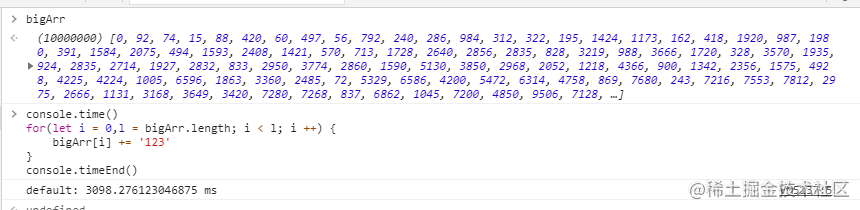## 理解 reduce

``````const arr = Array.from({length: 10}, (item, index) => ~~(Math.random()*100) * index)

const result = arr.reduce((acc, cur, index, source) => {
return acc
}, defaultValue)

### reduce 应该是个数据生成器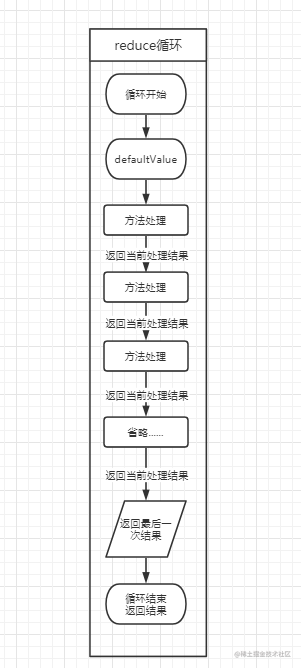### 从 polyfill 去理解

``````if (!Array.prototype.reduce) {
Object.defineProperty(Array.prototype, 'reduce', {
value: function(callback) {
var o = Object(this);
var len = o.length >>> 0;
var k = 0;
var value = arguments;
while (k < len) {
if (k in o) value = callback(value, o[k], k, o);
k++;
}
return value;
}
});
}

MDN 中 reduce 的部分 polyfill 代码(断言部分我们就不摘出来了)

## reduce 使用场景

### 生成 Object

#### ①数据归类

``````const data = [1,2,3,'a','b','c',[1,2,3],['a','b','c'],{a:1},{b:2},{c:3}]

function classify (data) {
return Object.prototype.toString.call(data).slice(8, -1)
}

const result = data.reduce((acc,cur) => {
const className = classify(cur)
acc[className] ? acc[className].push(cur) : acc[className] = [cur]
return acc
}, {})

console.log(result)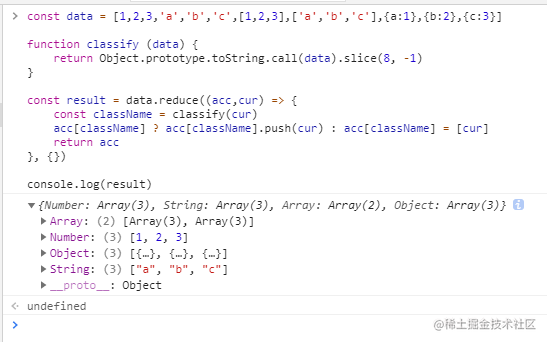#### ②生成数据字典

``````const data = [
{ name: 'Alice', id: 1 },
{ name: 'Max', id: 2 },
{ name: 'Jane', id: 3 },
{ name: 'roxz', id: 4 },
{ name: 'zonby', id: 5 },
{ name: 'zark', id: 6 },
];
const dataMap = data.reduce((acc, cur) => (acc[cur.id] = cur, acc), {})

console.log(dataMap)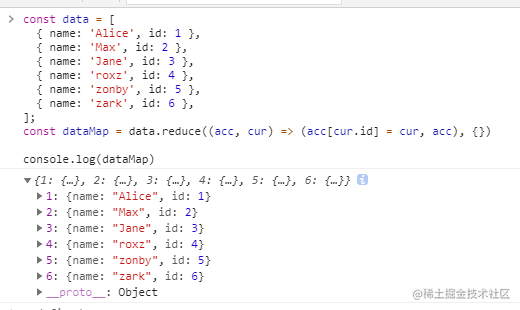### 生成 String

``````const data = ['a','b','d','h',123,'x']
function dispatchString (string) {
switch(data) {
case 'a':
return 'abc'
case 'd':
return 'def'
case 'x':
return 'xyz'
default:
return '|'
}
}

const result = data.reduce((acc,cur) => acc += dispatchString(cur), '')

console.log(result)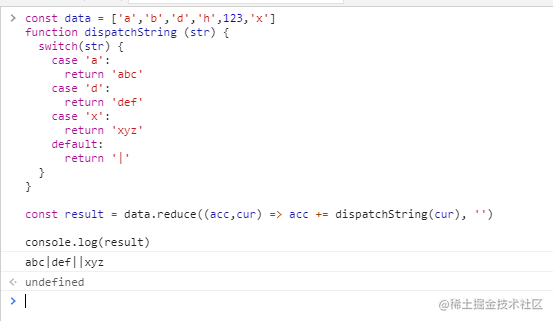### 生成 Array

``````  const data = [1,2,3,4,5,6,7,8,9]
// 数组正常处理
const mapFilterResult = data.map(item => ~~(Math.random()*100) * item).filter(item => item > 50)
// reduce 处理
const reduceResult = data.reduce((acc, cur) => {
const data = ~~(Math.random()*100) * cur
if (data <= 50) return acc // <= 50 直接跳过进入下个步骤
acc.push(data) // >50 保留下来
return acc
}, [])

console.log(mapFilterResult)
console.log(reduceResult)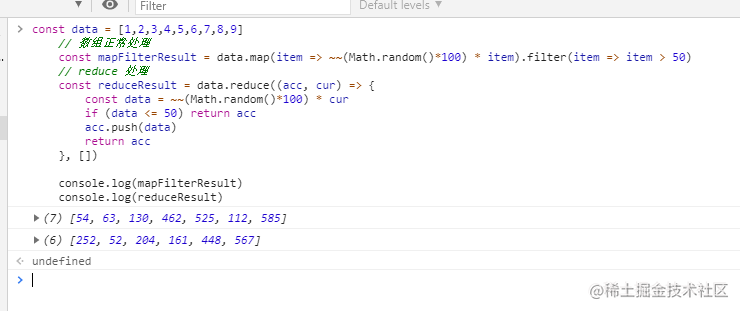## reduce 的限制

``````const data = [1,2,3,4,5,6,7,8,9,10]

const result = data.map((item, index) => {
if (item > 5) item = data //
return item * index
})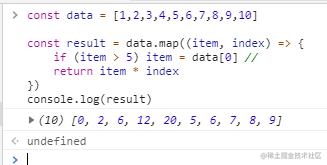``````const data = [1,2,3,4,5,6,7,8,9,10]

const result = data.reduce((acc, cur, index) => {
if (cur > 5) cur = acc
acc.push(cur * index)
return acc
}, [])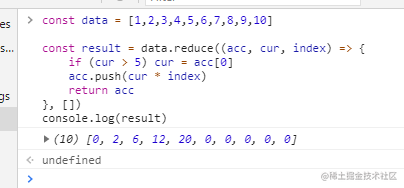acc 为处理后的值，依次为 0 * 5/6/7/8/9